﻿ 基于车牌信息的车辆出行信息分析系统设计——以桂林市为例 Design of Vehicle Travel Information Analysis System Based on License Plate Information—Case Study in Guilin

Open Journal of Transportation Technologies
Vol. 08  No. 06 ( 2019 ), Article ID: 32855 , 12 pages
10.12677/OJTT.2019.86045

Design of Vehicle Travel Information Analysis System Based on License Plate Information

—Case Study in Guilin

Liangyu Liu, Chen Gan, Tao Wang*, Wenyong Li

School of Architecture and Transportation Engineering, Guilin University of Electronic Technology, Guilin Guangxi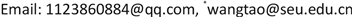Received: Oct. 18th, 2019; accepted: Oct. 31st, 2019; published: Nov. 7th, 2019ABSTRACT

License plate recognition technology provides a convenient and efficient data source for data mining and application of traffic survey. This system is based on the license plate information acquired by the measuring points in the city. According to the demand of traffic characteristic analysis, this system mainly uses Python and SQL to carry out relevant programs’ designing and data visualization processing. It realizes the generation of vehicle path, the acquisition of vehicle OD distribution between measuring points, and the generation of vehicle travel time distribution curve between neighboring points and some other practical functions. By connecting with the database, the primitive data can be updated in time and processed in large quantities, which provides a quality data source for further analysis and research of vehicle travel characteristics, provides intuitive reference for traffic planning and management, and helps the designer and management departments to carry out analysis of traffic system and design of problem-solving measures more effectively, then can improve the operation of the transportation system.

Keywords:License Plate Information, Path Generation, OD Distribution, Visualization

——以桂林市为例1. 设计背景与目的

2. 设计方法与技术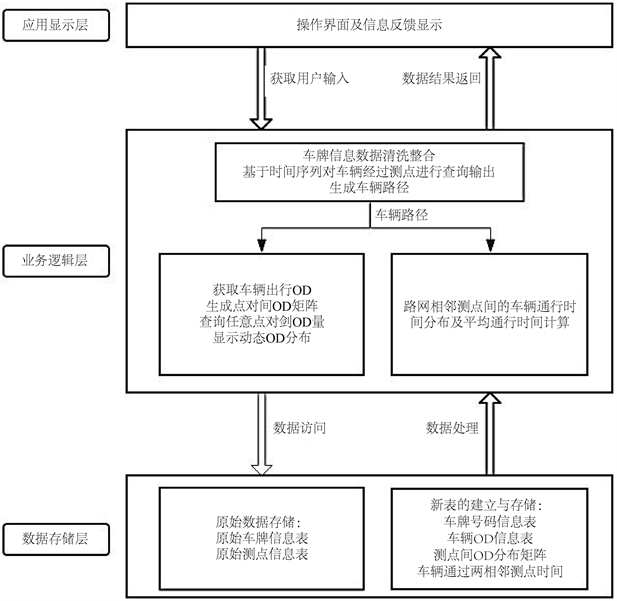Figure 1. Framework of system design

3. 数据清洗与整合

$\text{D}\left({\text{A}}_{\text{i}},{\text{B}}_{\text{j}}\right)=\left\{\begin{array}{c}0\text{\hspace{0.17em}}\text{\hspace{0.17em}}\text{\hspace{0.17em}}\text{\hspace{0.17em}}\text{\hspace{0.17em}}\text{\hspace{0.17em}}\text{\hspace{0.17em}}\text{\hspace{0.17em}}\text{\hspace{0.17em}}\text{\hspace{0.17em}}\text{\hspace{0.17em}}\text{i}=0,\text{j}=0\\ \mathrm{min}\left\{\begin{array}{c}{\text{d}}_{\text{k}-1}{\text{a}}_{\text{k}}={\text{b}}_{\text{k}}\\ {\text{d}}_{\text{k}-1}+1{\text{a}}_{\text{k}}\ne {\text{b}}_{\text{k}}\end{array}\end{array}$

$\text{R}=1-\frac{\text{D}\left({\text{A}}_{\text{i}},{\text{B}}_{\text{j}}\right)}{9}$

4. 系统功能及应用

4.1. 数据描述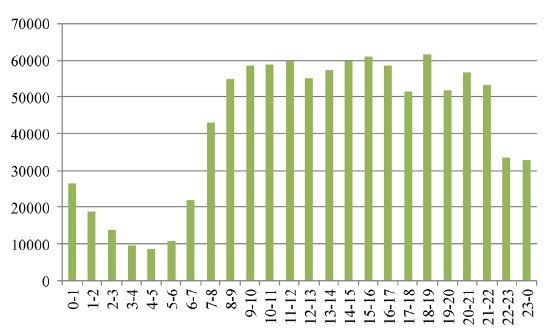Figure 2. Guilin vehicle travel time distribution composite

4.2. 车辆路径查询与生成

4.3. 相邻测点间通行时间分布曲线图绘制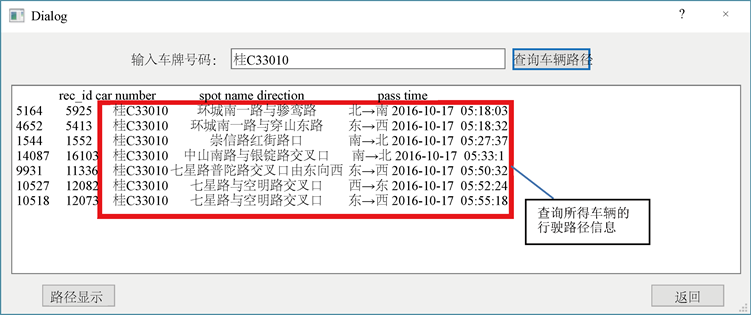Figure 3. Example of vehicle route query results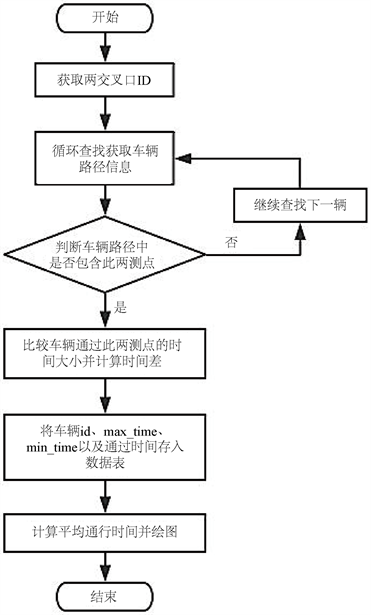Figure 4. Flowchart of calculating travel time and drawing distribution curve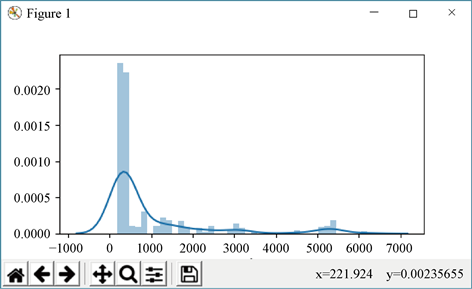Figure 5. Curve of vehicle travel time between sample measuring points

4.4. 测点间OD分布与矩阵生成

4.5. 实例应用与分析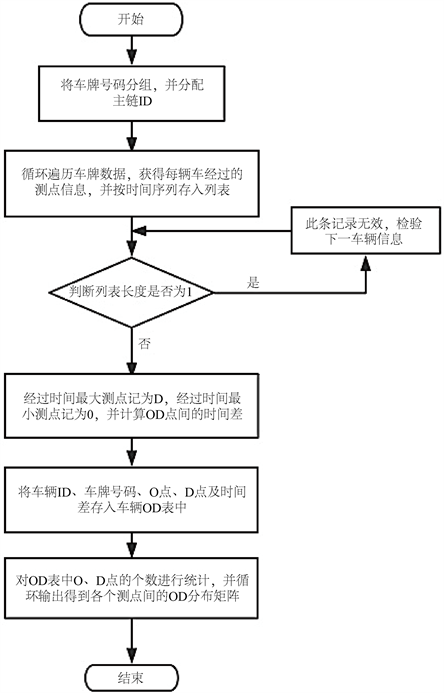Figure 6. Flowchart of acquisition of OD matrix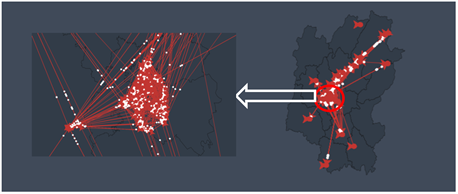Figure 7. Macroscopic and mesoscopic screenshots of dynamic OD distribution among measuring points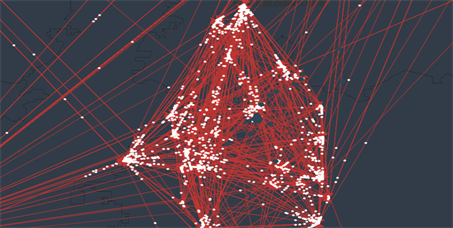Figure 8. Microscopic screenshots of dynamic OD distribution among measuring points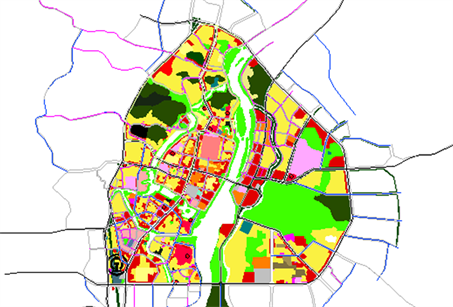Figure 9. Distribution of land use property and road network in the central area of Guilin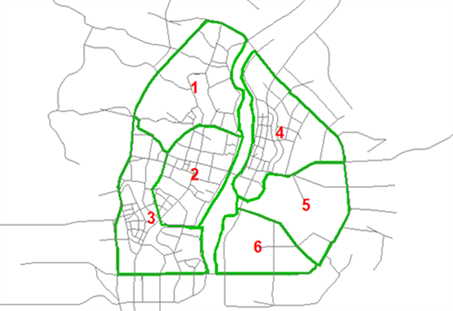Figure 10. Distribution of traffic district in the central area of GuilinTable 2. Measurement points and occurrence attraction in traffic districts (times/hour)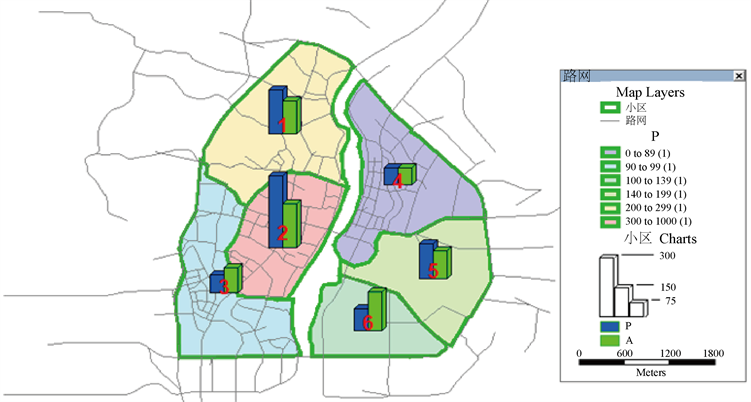Figure 11. Distribution of balanced traffic production and attraction in each district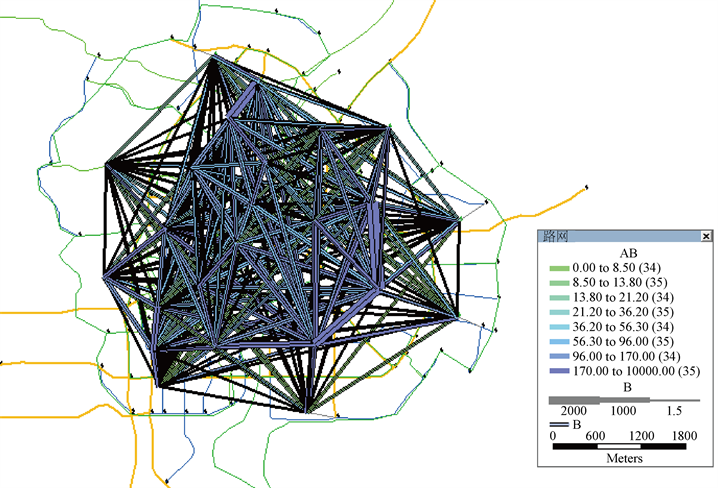Figure 12. OD expectation line for vehicle travel in the central area of Guilin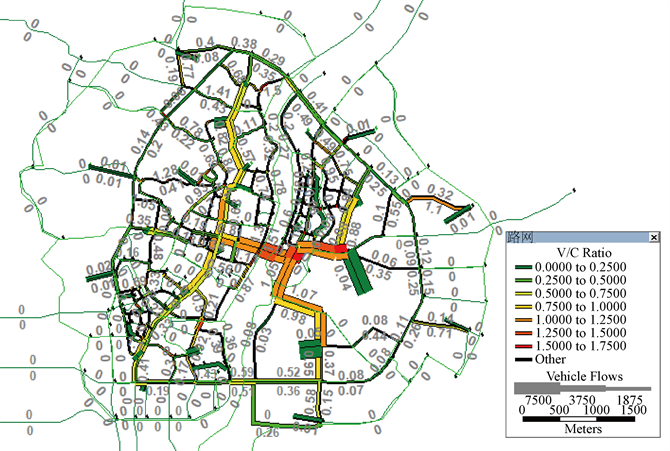Figure 13. Results of traffic distribution section in the central district of Guilin

5. 功能扩展

6. 总结与展望

Design of Vehicle Travel Information Analysis System Based on License Plate Information—Case Study in Guilin[J]. 交通技术, 2019, 08(06): 364-375. https://doi.org/10.12677/OJTT.2019.86045

1. 1. 郭昕, 陈川. 基于车牌识别数据的车辆使用特征研究: 以上海市快速路非沪车牌识别数据为例[J]. 综合运输, 2016(1): 77-84.

2. 2. 马金麟, 张宗博, 谢君平, 等. 基于车牌识别数据的车辆OD矩阵获取研究[J]. 重庆理工大学学报(自然科学), 2017, 31(7): 48-55.

3. 3. 胡旭峰. 基于车牌识别数据的城市道路交通状态判别及旅行时间可靠性的研究[D]: [硕士学位论文]. 青岛: 青岛科技大学, 2017.

4. 4. 刘静. 基于模糊匹配的多级车牌匹配技术[J]. 现代电子技术, 2015(7): 135-138.

5. 5. 王亚群. 基于车牌识别数据的城市路网运行状态识别方法实践[J]. 交通与运输(学术版), 2018(z1): 94-98.

6. NOTES

*通讯作者。